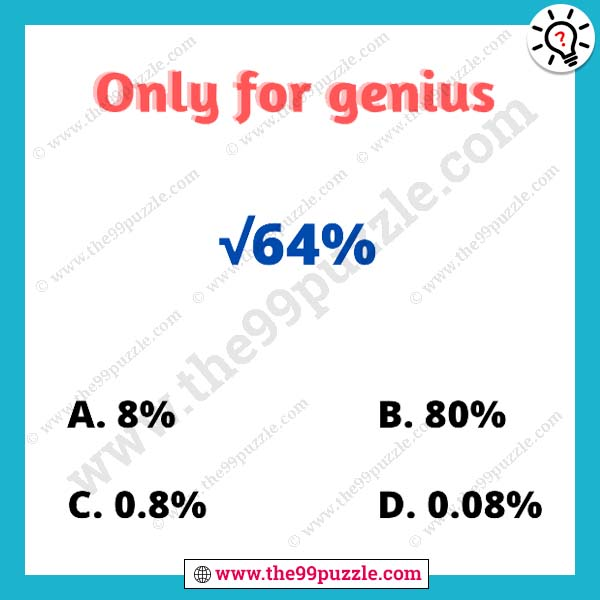# Easy and challenging math puzzle for genius – Puzz252

90% of genius people fail to give the correct answer. Can you solve the mathematics problem? This math asks in many competitive exams and interviews. Solve the math and choose the right answer. All school students accept these types of math puzzle challenges. Easy and challenging math puzzle for genius. This fun and logical math puzzle make you smarter if you practice every day. In this mathematics, you have to solve the math and give the correct answer. Most genius students solve math puzzles very quickly if you are a genius at puzzles you can solve this.√64%

⇒ √64/100

⇒ √8×8 / 10×10

⇒ 0.8×100 = 80%